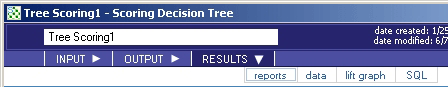# 5.4.5 - Tree Scoring - RESULTS - Reports - Teradata Warehouse Miner

## Teradata Warehouse Miner User Guide - Volume 3Analytic Functions

Product
Release Number
5.4.5
Published
February 2018
Language
English (United States)
Last Update
2018-05-04
dita:mapPath
yuy1504291362546.ditamap
dita:ditavalPath
ft:empty
dita:id
tbj1499730075274
1. On the Tree Scoring dialog box, click RESULTS.
2. Click reports (note that the RESULTS tab is disabled until after the analysis is completed).
Tree Scoring > Results > Reports• Decision Tree Score Report
• Resulting Scored Table Name — This is the name given the table with the scored values of the decision tree model.
• Number of Rows in Scored Table — This is the number of rows in the scored decision tree table.
• Confusion Matrix — A N x (N+2) (for N outcomes of the dependent variable) confusion matrix is given with the following format.
Confusion Matrix
Actual ‘0’ Actual ‘1’ Actual ‘N’ Correct Incorrect
Predicted ‘0’ # correct ‘0’ Predictions # incorrect‘1’ Predictions # incorrect ‘N’ Predictions Total Correct ‘0’ Predictions Total Incorrect ‘0’ Predictions
Predicted ‘1’ # incorrect‘0’ Predictions # correct ‘1’ Predictions # incorrect ‘N’ Predictions Total Correct ‘1’ Predictions Total Incorrect ‘1’ Predictions
Predicted ‘N’ # incorrect‘0’ Predictions # incorrect ‘1’ Predictions # correct ‘N’ Predictions Total Correct ‘N’ Predictions Total Incorrect ‘N’ Predictions
• Cumulative Lift Table — The Cumulative Lift Table demonstrates how effective the model is in estimating the dependent variable. It is produced using deciles based on the probability values. Note that the deciles are labeled such that 1 is the highest decile and 10 is the lowest, based on the probability values calculated by logistic regression. The information in this report, however, is best viewed in the Lift Chart produced as a graph. This is only valid for binary dependent variables.
• Decile — The deciles in the report are based on the probability values predicted by the model. Note that 1 is the highest decile and 10 is the lowest. That is, decile 1 contains data on the 10% of the observations with the highest estimated probabilities that the dependent variable is 1.
• Count — This column contains the count of observations in the decile.
• Response — This column contains the count of observations in the decile where the actual value of the dependent variable is 1.
• Pct Response — This column contains the percentage of observations in the decile where the actual value of the dependent variable is 1.
• Pct Captured Response — This column contains the percentage of responses in the decile over all the responses in any decile.
• Lift — The lift value is the percentage response in the decile (Pct Response) divided by the expected response, where the expected response is the percentage of response or dependent 1-values over all observations. For example, if 10% of the observations overall have a dependent variable with value 1, and 20% of the observations in decile 1 have a dependent variable with value 1, then the lift value within decile 1 is 2.0, meaning that the model gives a “lift” that is better than chance alone by a factor of two in predicting response values of 1 within this decile.
• Cumulative Response — This is a cumulative measure of Response, from decile 1 to this decile.
• Cumulative Pct Response — This is a cumulative measure of Pct Response, from decile 1 to this decile.
• Cumulative Pct Captured Response — This is a cumulative measure of Pct Captured Response, from decile 1 to this decile.
• Cumulative Lift — This is a cumulative measure of Lift, from decile 1 to this decile.